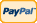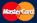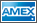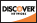•Electronics, electrical engineering
•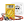Engineering physics
•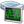Generators
•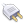Interfaces
•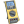Multimeters
•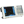Oscilloscopes
•Persona
•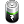Power supplies
•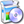Software
•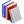Standards
•Thesaurus
•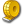Units of measurement
Filter by first letter
A
B
C
D
E
F
G
H
I
J
K
L
M
N
O
P
Q
R
S
T
U
V
W
X
Y
Z
+
-

# Sample Rate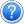The sampling rate, sample rate, or sampling frequency defines the number of samples per unit of time (usually seconds) taken from a continuous signal to make a discrete signal. For time-domain signals, the unit for sampling rate is hertz (inverse seconds, 1/s, s−1), sometimes noted as Sa/s (samples per second). The inverse of the sampling frequency is the sampling period or sampling interval, which is the time between samples.

For digital scopes, sampling rate and memory depth are equally important. For DSOs, the sampling rate is usually specified in mega samples per second (MS/s) or gigasamples per second (GS/s). The Nyquist criterion states that the sampling rate must be at least twice the maximum frequency that you want to measure: for a spectrum analyser this may be true, but for a scope you require at least 5 samples to accurately reconstruct a waveform.

Most scopes have two different sampling rates (modes) depending on the signal being measured: realtime and equivalenttime sampling (ETS)  often called repetitive sampling. However, ETS only works if the signal you are measuring is stable and repetitive, since this mode works by building up the waveform from successive acquisitions.

Some scopes have different sampling rates, depending on the number of channels in use. Typically, the sampling rate in single channel mode is twice that in dual channel mode.

Oversampling

In some cases it is desirable to have a sampling frequency more than twice the desired system bandwidth so that a steep digital filter and a less steep analog anti-aliasing filter can be used in exchange for a steep analog anti-aliasing filter. The reason for wanting a less steep analog anti-aliasing filter is that the digital filter is not subject to any component variations thus always giving the filter response (filtering function) that the designer has chosen. This process is known as oversampling.

Undersampling

Conversely, one may sample below the Nyquist rate. For a baseband signal (one that has components from 0 to the band limit), this introduces aliasing, but for a passband signal (one that does not have low frequency components), there are no low frequency signals for the aliases of high frequency signals to collide with, and thus one can sample a high frequency (but narrow bandwidth) signal at a much lower sample rate than the Nyquist rate.

Sources: www.picotech.com, www.wikipedia.org

Back to the list

Units Converter
• How it Works
• Company News
• Industry News & Events
• Measurement Fun FactsSite map|Privacy policy|Terms of Use & Store Policies|How to Buy|Shipping|Payment|© T&M Atlantic, Inc., 2010-2022